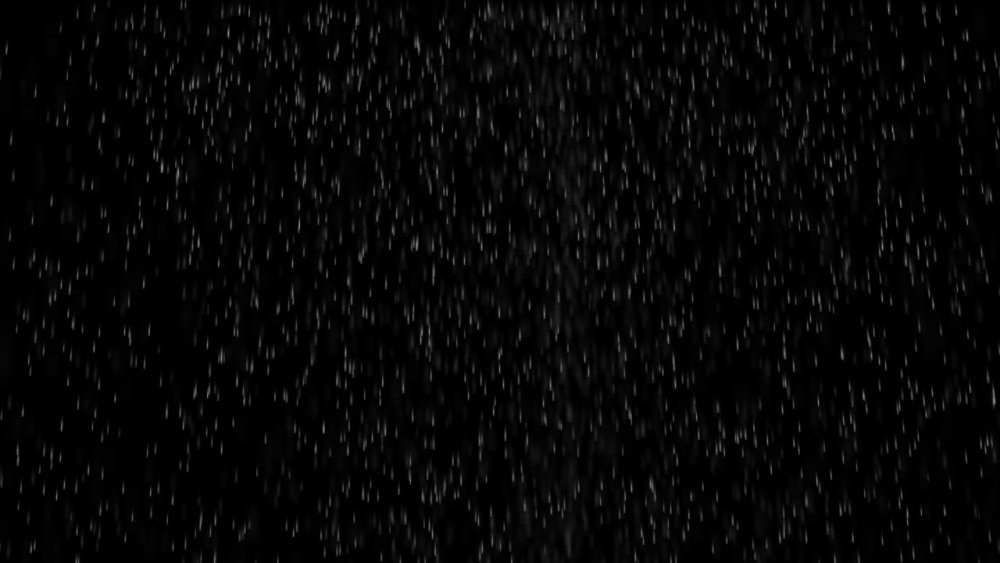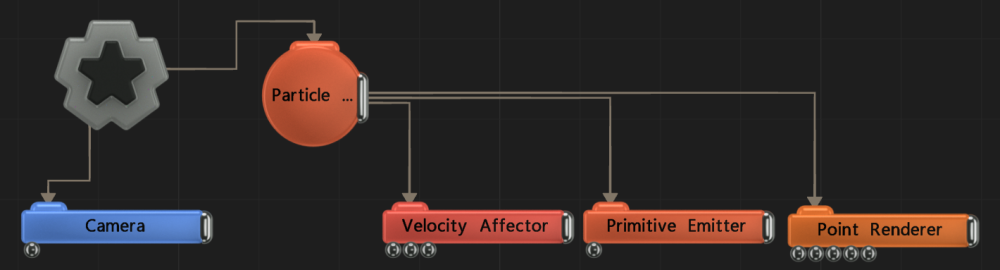## Method

This node applies a constant velocity to the affected particles. This is typically used to make particles move in a regular, linear fashion, e.g. wind effects or repulsions.

The Mode attribute is used to determine if the velocity is directional with a random spread determined by the Spread Angle attribute, or if it is an attracting or repelling velocity generated from the effector’s position. The magnitude of the velocity is controlled by the Velocity Scale attribute and modulated by a random value which is constant per particle and weighted by the Randomness attribute.

The velocity effect is weighted by a falloff calculated using the distance from the node to the particle. The effect is at 100% up to the distance specified by the Inner Radius attribute, then ramps down to 0% at the distance specified by the Radius attribute.

ExampleThis image is taken from the “simple_rain.dfx” sample project.
Example Nodegraph## Transform

Name Description
Position X Move its position along the x-axis in local space.
Position Y Move its position along the y-axis in local space.
Position Z Move its position along the z-axis in local space.
Rotation Pitch Rotate the object about the y-axis.
Rotation Bank Rotate the object about the z-axis.
Scale X Scale along the x-axis.
Scale Y Scale along the y-axis.
Scale Z Scale along the z-axis.

## Inherit Transform Channels

Toggle which transform channels should be inherited. By default, all are on.

## Attributes

Name Description
Velocity Amount Scale the strength of the affectors velocity on the particles.
Radius Alter the outer radius at which the affector is no longer effective.
Inner Radius Alter the inner radius up to which the affector is fully effective.
Randomness How much randomness is added in the particles movement.
Mode Choose what kind of velocity affects the particles.
Falloff Shape The primitive shape used to calculate the falloff weight for the affector.
• Point, uses the distance from the centre position of the node
• Line, uses the distance from an infinite line in the X axis centred at the position of the node
• Plane, uses the distance from an infinite plane in the XY axis centred at the position of the node.
Life Effect Coeffs How much the particles are affected by the affector at different stages of the particles life cycle. Values 1 and 2 are control points used to control a bezier curve between values 0 and 3.

## Inputs

Name Description Typical Node Input
Velocity Node A transform node that overrides the velocity direction and source position. Null